Courses

# Test: Introduction & Properties of Fluid Level- 2

## 15 Questions MCQ Test Engineering Mechanics | Test: Introduction & Properties of Fluid Level- 2

Description
This mock test of Test: Introduction & Properties of Fluid Level- 2 for Civil Engineering (CE) helps you for every Civil Engineering (CE) entrance exam. This contains 15 Multiple Choice Questions for Civil Engineering (CE) Test: Introduction & Properties of Fluid Level- 2 (mcq) to study with solutions a complete question bank. The solved questions answers in this Test: Introduction & Properties of Fluid Level- 2 quiz give you a good mix of easy questions and tough questions. Civil Engineering (CE) students definitely take this Test: Introduction & Properties of Fluid Level- 2 exercise for a better result in the exam. You can find other Test: Introduction & Properties of Fluid Level- 2 extra questions, long questions & short questions for Civil Engineering (CE) on EduRev as well by searching above.
QUESTION: 1

### In general decrease in temperature, results in

Solution: Viscosity of liquids depends upon inter molecular cohesion which weakens when temperature is raised, hence decreasing the viscosity whereas the viscosity of gases depends predominantly on inter molecular momentum transfer by collision, which increases with temperature, hence increasing the viscosity of gases. So with a decrease of temperature, the viscosity of liquids increases whereas the viscosity of gases decreases.

QUESTION: 2

### What is the radius of Mohr’s stress (in kPa) circle for a point inside a fluid mass which rotates like a solid body in a cylindrical container. The pressure at the point is 200 kPa.

Solution: For a fluid mass which moves as a solid body, the shear stress at any plane passing through any point is zero. Hence, the stress on all the planes passing through a point is equal. In such a condition Mohr’s circle is a point on the normal stress axis (−ve) and the distance of the point from origin is equal to pressure at the point.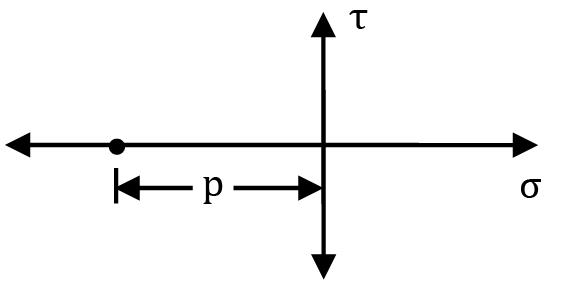QUESTION: 3

### Which one of the following is the bulk modulus K of a fluid? (Symbols have the usual meaning)

Solution: For any fluid particle m = ρV As this fluid particle will experience a change in pressure, it’s ρ and V will change but m will remain constant.

∴ dm = d(ρV)

0 = ρdV + Vdρ

−dV / V = dp / ρ

As per definition of Bulk Modulus

K = dp / (−dV / V)

Therefore

K = dp / dρ/ρ

K = ρ dp / dρ

QUESTION: 4

If angle of contact of a drop of liquid is acute, then

Solution: If contact angle of liquid is acute, that means the liquid can easily wet the surface i.e., the force of adhesion between the liquid and surface is stronger than the cohesive forces acting between the molecules of the liquid. Example: water

QUESTION: 5

Which of the following fluids exhibit a certain shear stress at zero shear strain rate followed by a straight line relationship between shear stress and shear strain rate?

Solution: The shear stress vs. rate of strain behavior of different fluids is as shown in the figure.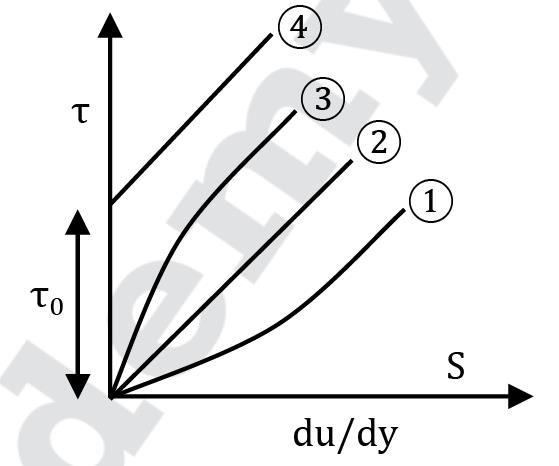Where

1 → Dilatants like quicksand, oobleck

2 → Newtonian fluids like water, air

3 → Pseudo plastics like blood, milk

4 → Bingham substances like mayonnaise, toothpaste

Bingham substances are those which undergo static deformation upto a limiting value (τ) of shear stress and behave as solids but beyond this limiting value they deform continuously and behave as fluids.

Thus if τ < τ0,="" even="" though="" there="" will="" be="" deformation="" but="" the="" rate="" of="" deformation="" will="" be="" />

QUESTION: 6

An increase in pressure of a liquid from 7.5 MPa to 15 MPa results into 0.2 percent decrease in its volume. The coefficient of compressibility of the liquid in m2/N is

Solution: Given p2 = 15 × 106 Pa p1 = 7.5 × 106 Pa

ΔV / V× 100 = −0.2

⇒ ΔV / V= −2 × 10-3

K = Δp / −Δ? / ?

= (15 − 7.5) × 106 / −(−2 × 10−3)

= 3.75 × 109

Compressibility = 1 / K = 2.666 × 10-10

≃ 0.267 × 10−9 m2/N

QUESTION: 7

If the surface tension of water-air interface is 0.073 N/m, the gauge pressure inside a rain drop of 1 mm diameter will be

Solution: Cut the raindrop in two halves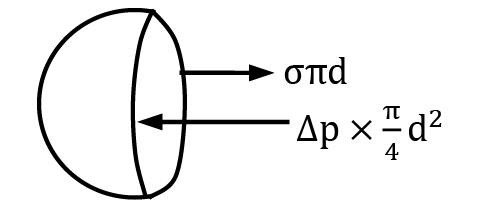For the raindrop to be in equilibrium,

σπd = Δp × π / 4 d2

4σ d = Δp

Substituting values,

Δp = 4 × 0.073 / 1 × 10-3

Δp = 292 N/m2

QUESTION: 8

For the figure shown below, match List-I (curve) with List-II (type of fluid).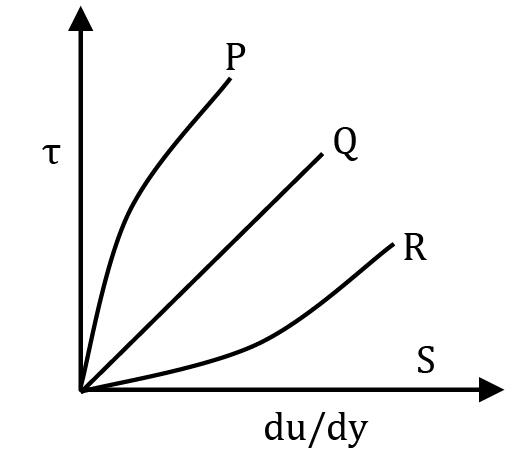List-I (Curve) List-II (Type of fluid)

a. P 1. Newtonian fluid

b. Q 2. Pseudo plastic fluid

c. R 3. Dilatants

d. S 4. Bingham fluids

5. Ideal solid

6. Ideal fluids

Codes: a b c d

Solution:
QUESTION: 9

The vapour pressure is the characteristic fluid property involved in the phenomenon of

Solution: If pressure at a point in a flow field drops below the vapor pressure of the liquid, bubble formation will occur at the point. As these bubbles move ahead into high pressure zone, they burst creating turbulence. As a result pitting and corrosion occurs on the nearby solid surfaces.

QUESTION: 10

The absolute pressure inside a liquid jet is slightly greater than the atmospheric pressure. This excess pressure (p), the surface tension (σ) of the liquid and diameter (d) of jet are related as

Solution: Cut the liquid jet into two halves and consider FBD of one half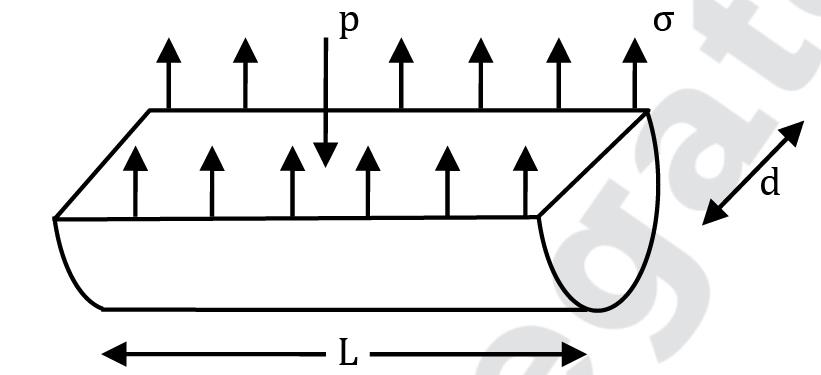⇒ p × (Ld) = σ × 2L

⇒ p = 2σ / d

QUESTION: 11

The Mohr’s stress circle for any point considered inside a fluid at rest is given as

Solution:

For a fluid at rest, shear stress at any plane passing through a point is zero hence all the planes are subjected to equal normal stress (pressure). Thus Mohr’s circle is a point on the −ve σ axis. And the distance of the point from origin is equal to pressure.

QUESTION: 12

With increase in pressure, the bulk modulus of elasticity for an ideal gas

Solution: Consider an ideal gas initially at a state given by (p, V, T) If the ideal gas undergoes a polytropic process given by pVn = C, an infinitesimal change in state can be given as

d(pVn) = 0

⇒ pnVn−1 dV + dp Vn = 0

⇒ dp = − 1 / npdV − np dV / V

⇒dp / (−dV / V) = np

⇒ K = np

Thus Bulk modulus of an ideal gas is directly dependent upon pressure i.e., if p ↑ K ↑

QUESTION: 13

Assertion (A): An open glass tube is dipped in mercury. The mercury level inside the tube shall rise above the level of mercury outside.

Reason (R): The cohesive force between the molecules of mercury is greater than the adhesive force between mercury and glass.

Solution: When a glass tube is introduced into mercury, the level of mercury drops inside the glass tube the cohesive forces within the mercury molecules pull the mercury down along with formation of convex meniscus. Whereas in case of water, the adhesive force between the glass and water is strong and pulls the water upward forming concave meniscus. This capillary rise or depression is because of the difference of cohesive and adhesive forces of liquids for certain surfaces and not because of their density.

QUESTION: 14

Which of the following dimensionless no. represents applicability of concept of continuum analysis of a system?

Solution:

Knudsen number is the ratio of the molecular mean free path length to a representative physical length scale.

QUESTION: 15

The density of water is maximum at a temperature of

Solution:

For any liquid the density of the liquid decreases with increasing temperature. But water is an exception and shows maximum density at 4.4°C.